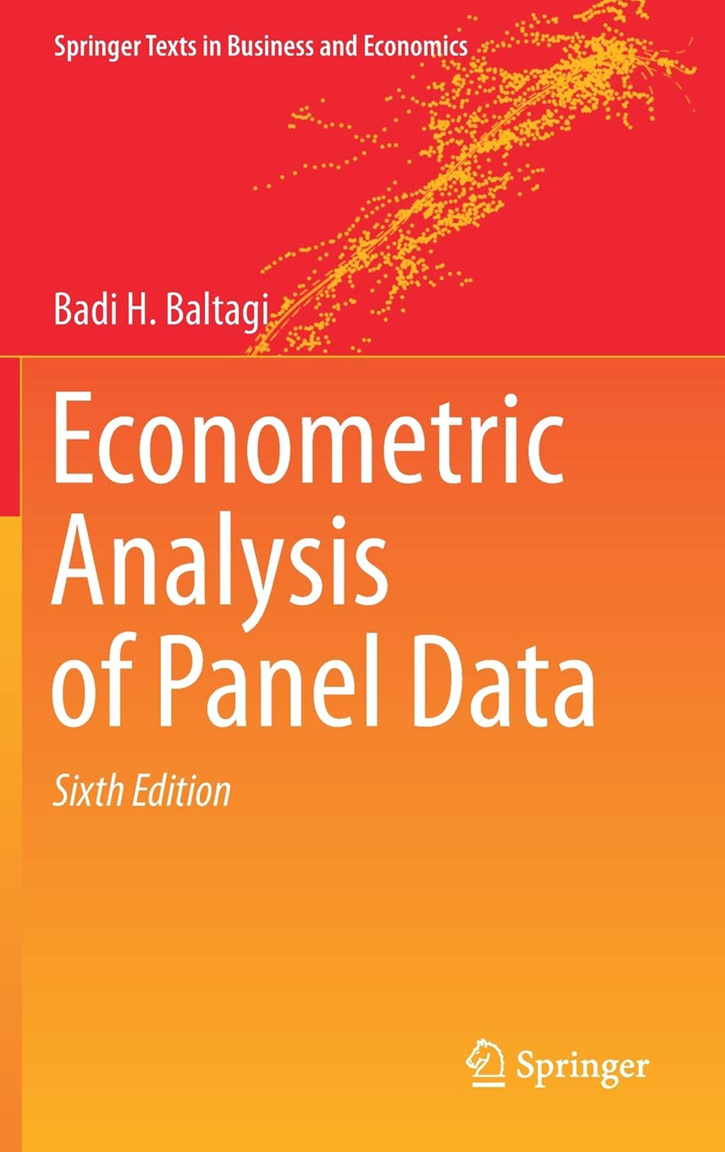# Econometric Analysis of Panel Data

Econometric Analysis of Panel Data, Sixth Edition, by Badi H. Baltagi, is a standard reference for performing estimation and inference on panel datasets from an econometric standpoint. This book provides both a rigorous introduction to standard panel estimators and concise explanations of many newer, more advanced techniques.

This book provides an excellent introduction for the student or the applied researcher because of its attention to detail and its use of examples, many of which use Stata. The detail is especially useful in the many sections that grow out of Baltagi’s own work. In these sections, readers gain a deep enough understanding of the models to implement them in a programming language like Stata. In other sections, such as the chapter on limited dependent variables, Baltagi combines a good introduction to the mechanics with an excellent introduction to the literature, allowing readers the opportunity to follow up for more details.

The sixth edition has been substantially updated to reflect modern developments in panel-data analysis. This edition also includes new material on dynamic panels, limited dependent variables, nonstationary panels, and spatial panel data.

Because of its wide range of topics and detailed exposition, Econometric Analysis of Panel Data, Sixth Edition can serve as both a graduate-level textbook and a handy desk reference for seasoned researchers.

INTRODUCTION

Panel Data: Some Examples

Examples of Micro-panels
Examples of Macro-panels
Some Basic References

Why Should We Use Panel Data? Their Benefits and Limitations
Note

THE ONE-WAY ERROR COMPONENT REGRESSION MODEL

Introduction
The One-Way Fixed Effects Model
The One-Way Random Effects Model
Maximum Likelihood Estimation
Prediction
Examples

Example 1: Investment Equation
Example 2: Gasoline Demand Equation
Example 3: Public Capital Productivity

Selected Applications
Computational Note
Notes
Problems

THE TWO-WAY ERROR COMPONENT REGRESSION MODEL

Introduction
The Two-Way Fixed Effects Model

Testing for Fixed Effects
The Two-Way Random Effects Model
Monte Carlo Results

Maximum Likelihood Estimation
Prediction
Examples

Example 1: Investment Equation
Example 2: Gasoline Demand Equation
Example 3: Public Capital Productivity

Computational Note
Notes
Problems

TEST OF HYPOTHESES WITH PANEL DATA

Tests for Poolability

Test for Poolability u~N(0,σ2INT)
Test for Poolability Under the General Assumption u~N(0,Ω)
Examples4.2 Tests for Individual and Time Effects
The Breusch—Pagan Test
Honda, King and Wu, and the Standardized Lagrange Multiplier Tests
Gourieroux, Holly and Monfort Test
Conditional LM Tests
ANOVA F and the Likelihood Ratio Tests
Monte Carlo Results
An Illustrative Example

Hausman’s Specification Test

Example 1: Investment Equation
Example 2: Gasoline Demand Equation
Example 4: Sawmills in Washington State
Example 6: Currency Union
Hausman’s Test for the Two-Way Model

Notes
Problems

HETEROSKEDASTICITY AND SERIAL CORRELATION IN THE ERROR COMPONENT MODEL

Heteroskedasticity

Testing for Homoskedasticity in an Error Component Model

Serial Correlation

The AR(1) Process
The AR(2) Process
The AR(4) Process for Quarterly Data
The MA(1) Process
Unequally Spaced Panels with AR(1) Disturbances
Prediction
Testing for Serial Correlation and Individual Effects

Time-Wise Autocorrelated and Cross-Sectionally Heteroskedastic Panel Regression
Notes
Problems

SEEMINGLY UNRELATED REGRESSIONS WITH ERROR COMPONENTS

The One-Way Model
The Two-Way Model
Applications and Extensions
Problems

SIMULTANEOUS EQUATIONS WITH ERROR COMPONENTS

Single Equation Estimation
Empirical Example: Crime in North Carolina
System Estimation
The Hausman and Taylor Estimator
Empirical Example: Earnings Equation Using PSID Data
Notes
Problems

DYNAMIC PANEL DATA MODELS

Introduction
The Arellano and Bond Estimator

Testing for Over-Indentification Restrictions and Serial Correlation in Dynamic Panel Models
Downward Bias of the Estimated Asymptotic Standard Errors
Too Many Moment Conditions and the Bias Efficiency Trade-Off

The Arellano and Bover Estimator
The Ahn and Schmidt Moment Conditions
The Blundell and Bond System GMM Estimator
The Keane and Runkle Estimator
Limited Information Maximum Likelihood
Empirical Examples

Example 1: Dynamic Demand for Cigarettes
Example 2: Democracy and Education

Selected Applications
Notes
Problems

UNBALANCED PANEL DATA MODELS

Introduction
The Unbalanced One-Way Error Component Model

ANOVA Methods

Maximum Likelihood Estimators

Minimum Norm and Minimum Variance Quadratic Unbiased Estimators (MINQUE and MIVQUE)
Monte Carlo Results

Empirical Example: Hedonic Housing
The Unbalanced Two-Way Error Component Model

The Fixed Effects Model
The Random Effects Model

Testing for Individual and Time Effects Using Unbalanced Panel Data
The Unbalanced Nested Error Component Model
Empirical Example: Nested States Public Capital Productivity
Notes
Problems

SPECIAL TOPICS

Measurement Error and Panel Data
Rotating Panels
Pseudo-Panels
Short-Run versus Long-Run Estimates in Pooled Models
Heterogeneous Panels
Count Panel Data
Notes
Problems

LIMITED DEPENDENT VARIABLES AND PANEL DATA

Fixed and Random Logit and Probit Models
Simulation Estimation of Limited Dependent Variable Models with Panel Data
Dynamic Panel Data Limited Dependent Variable Models
Selection Bias in Panel Data
Censored and Truncated Panel Data Models
Empirical Applications
Empirical Example: Nurses Labor Supply
Notes
Problems

NONSTATIONARY PANELS

Introduction
Panel Unit Roots Tests Assuming Cross-Sectional Independence

Levin, Lin and Chu Test
Im, Pesaran and Shin Test
Breitung’s Test
Combining p-Value Tests
Residual-Based LM Test

Panel Unit Roots Tests Allowing for Cross-Sectional Dependence
Spurious Regression in Panel Data
Panel Cointegration Tests

Residual-Based DF and ADF Tests (Kao Tests)
Residual-Based LM Test
Pedroni Tests
Likelihood-Based Cointegration Test
Finite Sample Properties

Estimation and Inference in Panel Cointegration Models
Empirical Examples

Example 2: International R&D Spillover
Example 3: OECD Health Care Expenditures

Notes
Problems

SPATIAL PANEL DATA MODELS

Introduction
Spatial Error Component Regression Model
Spatial Lag Panel Data Regression Model
Forecasts using Panel Data with Spatial Error Correlation
Panel Unit Root Tests and Spatial Dependence
Panel Data Tests for Cross-Sectional Dependence
Computational Note
Problems